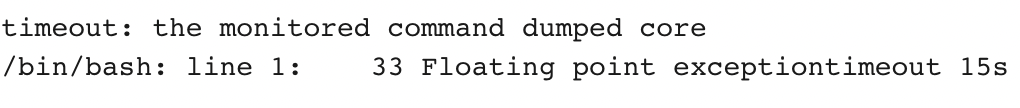Open in App
Not now

# Reason of runtime error in C/C++

• Difficulty Level : Easy
• Last Updated : 20 Dec, 2022

In this article, we will discuss the reason for the run-time error and its solution. Runtime Error: A runtime error in a program is an error that occurs while the program is running after being successfully compiled. Below are some methods to identify the reason behind Runtime errors:

Method 1: When the index of the array is assigned with a negative index it leads to invalid memory access during runtime error. Below is the C++ Program to illustrate the invalid memory access during run-time:

## C++

 `// C++ program to illustrate invalid``// memory access during run-time``#include ``using` `namespace` `std;` `// Global declaration``int` `arr;` `// Driver Code``int` `main()``{``    ``int` `answer = arr[-10];``    ``cout << answer;` `    ``return` `0;``}`

Output:

`1736487104`

Method 2: Sometimes Array or vector runs out of bounds of their limits resulting in a runtime error. Below is the C++ program illustrating array runs out of bound:

## C++

 `// C++ program to illustrate``// array runs out of bound``#include ``using` `namespace` `std;` `// Driver Code``int` `main()``{``    ``long` `n;``    ``n = 100000000000;` `    ``// 'n' is out of bound for``    ``// the array limit``    ``long` `a[n];` `    ``cout << a << ``" "``;``    ``return` `0;``}`

Explanation:

• This is an error for index out of bound.
• It can be resolved by using the size of the array/vector as within the limit.

Method 3: Some silly mistakes like dividing by zero encountered while coding in a hurry, sometimes leads to runtime error. Below is the C++ program illustrating runtime error by dividing by zero and un-assigned variables:

## C++

 `#include ``using` `namespace` `std;` `int` `main() {``      ``int` `n = 0;``    ``cout << 5/n;``    ``return` `0;``}`

Output:## C++

 `// C++ program to illustrate runtime``// error by un-assigned variables``#include ``using` `namespace` `std;` `// Driver Code``int` `main()``{``    ``long` `long` `N;` `    ``// N is assigned garbage value``    ``long` `arr[N];` `    ``cin >> N;` `    ``for` `(``int` `i = 0; i < N; i++) {``        ``cin >> arr[i];``    ``}` `    ``for` `(``int` `i = 0; i < N; i++) {``        ``cout << arr[i] << ``" "``;``    ``}` `    ``return` `0;``}`

Explanation:

The above program shows “Bad memory access (SIGBUS)” because:

• Here, variable N is assigned a garbage value resulting in a runtime error.
• Sometimes, it depends on how the compiler assigned the garbage value.
• This can be resolved by declaring arr[N] after scanning the value for variable n and checking if it is the upper or lower limit of the array/vector index.

My Personal Notes arrow_drop_up